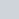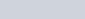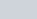A tree breaks due to the storm and the broken part bends so that the top
Question:

A tree breaks due to the storm and the broken part bends so that the top of the tree touches the ground making an angle of 30° with the ground. The distance from the foot of the tree to the point where the top touches the ground is 10 metres. Find the height of the tree.

Solution:

Let AB be the tree of height h. And the top of tree makes an angle 30° with ground. The distance between foot of tree to the point where the top touches the ground ism. Let BC = 10. And.

Here we have to find height of tree.

Here we have the corresponding figureSo we use trigonometric ratios.

In a triangle,

$\Rightarrow \quad \tan C=\frac{A B}{B C}$

$\Rightarrow \quad \tan 30^{\circ}=\frac{A B}{B C}$

$\Rightarrow \quad \frac{1}{\sqrt{3}}=\frac{h}{10}$

$\Rightarrow \quad h=\frac{10}{\sqrt{3}}$

Now in triangle ABC we have

$\sin 30^{\circ}=\frac{h}{A C}$

$\Rightarrow \frac{1}{2}=\frac{10}{\sqrt{3} A C}$

$\Rightarrow A C=\frac{20}{\sqrt{3}}$

So the length of the tree is

$=A B+A C$

$=h+A C$

$=\frac{10}{\sqrt{3}}+\frac{20}{\sqrt{3}}$

$=10 \sqrt{3}$

$=1.73$

Hence the height of tree is $17.3 \mathrm{~m}$.